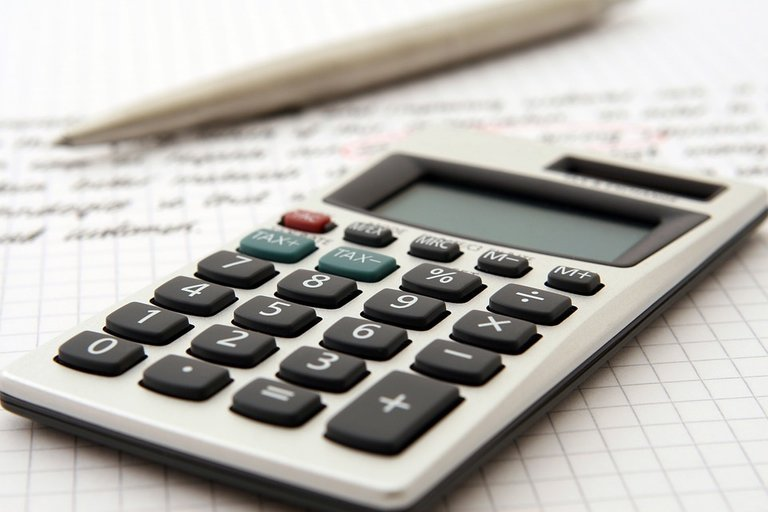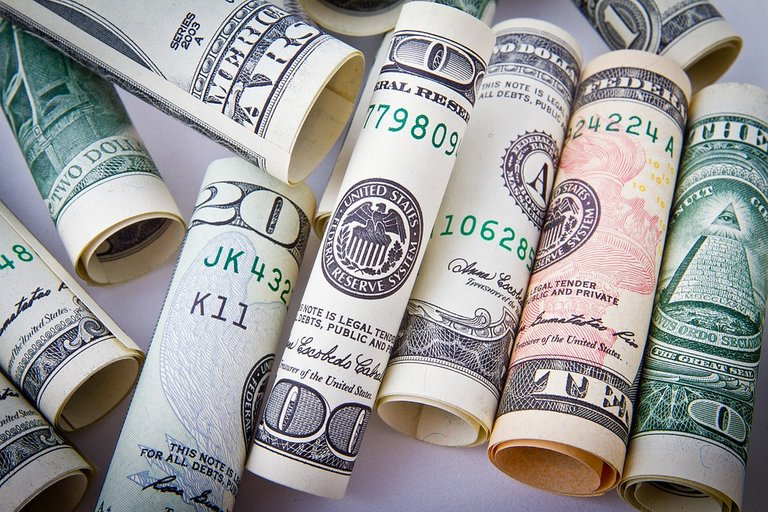# Breaking Even With Math Examples

in LeoFinancelast year

Hi there. In this post, I would like to show case math examples when it comes to breaking even on finance trades and investments.Pixabay Image Source

## Topics

• Review Of Percents
• Breaking Even Math Examples
• Is It Possible To Break Even?

## Review Of Percents

Percents refer to a part of a whole. One percent refers to one for each hundred or one out a hundred.

$$1% = \dfrac{1}{100} = 0.01$$

Common percents include 10%, 20%, 25%, 50%, 100%. You do get a few cases like 200% which represents 200 for each 100 or double and something like 1000% which is 10 times the original amount.

## Breaking Even Math Examples

One of the most awful feelings in life is losing money. There is a nautral need to win money back after losing money. You do have to be careful with trying to win money back in the stock market, cryptocurrencies and investments. The additional funds being put in can be lost too.

I present some examples with math calculuations.

Example One

Brad lost 50% of the value in his one stock XYZ. The price he bought it at was $120. The price went down to$60.

This XYZ stock needs to go up by $60 to go back to$120. In order for this stock to go back to $120 XYZ stock needs to double from 60 dollars. Example Two Maria lost 80% of the value in her crypto holdings. She bought her crypto when it was valued at$2000. The crypto Maria holds is now $400 (retains 20% of value or lost$1600 in value).

For Maria to break even from $400 to$2000, she needs an increase of $1600 in her crypto holdings. This$1600 is four times $400. Maria would need her crypto to quadruple in value. Example Three - Extreme Case Lola lost 99% of the value in highly speculative memecoins. She put in$100 at first and now the value of the memecoins is $1. For Lola to break even back to$100, the memecoins price need to multiply by 100. This is highly unlikely.

General Formula

For those interested, there is a bit of a general formula for breaking even. Look into the amount retained in percentages terms. Do 1 divided by the percentage retained after the loss. (Reciprocal)

In the Maria example where she lost 80% of her holdings. As she lost 80% in value, she retained 20% of the value. After doing 1 divided by 20% this would be 5. Five as in Maria needs her crypto holdings to multiply 5 times in value from $400 to$2000. Technical calulations shown below.

$$1 \div 20% = 1 \div \dfrac{20}{100} = 1 \div \dfrac{1}{5} 1 \times \dfrac{5}{1} = 5$$
$$\text{General Formula: } 1 \div \text{Percentage Retained after Loss}$$Pixabay Image Source

## Is It Possible To Break Even?

After going through some of the examples in the previous section, you may ask yourself if it is even possible to break even after losses. A loss of 50% on an investment requires the investment to double to break even. A large loss of 90% of an investment would require the reduced price to multiply by ten times to get back to even. Is it realistic or even possible for something to multiply in price by ten? Be careful with buy entries.Pixabay Image Source